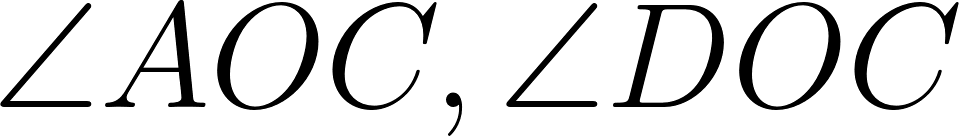Holt California Geometry - 1 Edition - Chapter 1-3 - Problem 29
Register Now

Join StudySoup

Get Full Access to Holt California Geometry - 1 Edition - Chapter 1-3 - Problem 299780030923456

# Find the value of x.mAOC = 7x - 2, mDOC = 2x + 8, mEOD = 27

Holt California Geometry | 1st Edition

Problem 29

Find the value of x.mAOC = 7x - 2, mDOC = 2x + 8, mEOD = 27

Accepted Solution
Step-by-Step Solution:

Step 1 of 2forms a straight line, so it is a straight angle and its measure isconsists ofand, so its measure is given by the sum of measures of the other three angles.###### Chapter 1-3, Problem 29 is Solved

Step 2 of 2

Unlock Textbook Solution